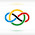Prove for all positive reals $a,b,c$,

$$\sum_{cyc} \frac{a}{\sqrt{3ab+bc}} \ge \frac 32.$$

I'll wait for comments and will post the solution in 2 days!

EDIT - Well now that it's been 3 days (oops i forgot to post), I'll show my solution now.

By Holder,$$\left( \sum_{cyc} \frac{a}{\sqrt{3ab+bc}} \right)^2 \left( \sum_{cyc} a(3ab+bc) \right) \ge (a+b+c)^3$$

So it suffices to show$$(a+b+c)^3 \ge \frac 94 \sum_{cyc} a(3ab+bc)$$

Expanding and cancelling terms, we wish to show$$f(a,b,c)=4\sum_{cyc} a^3 + 12\sum_{cyc} b^2a-15\sum_{cyc} a^2b-3abc\ge 0$$

Claim: $f(a,b,c) \le f(a+d, b+d, c+d)$ if $d>0$

Proof:$$f(a+d,b+d,c+d)-f(a,b,c) =d (4\sum_{cyc} 3a^2 + 12\sum_{cyc} (b^2+2ba) - 15\sum_{cyc} (a^2+2ba) - 3(ab+bc+ca))$$

$$+d^2 (4\sum_{cyc} 3a + 12 \sum_{cyc} (2b+a) - 15\sum_{cyc} (2a+b) - 3(a+b+c)$$

The $d^2, d^3$ stuff cancel. So $f(a+d,b+d,c+d) - f(a,b,c) = \frac{9d}{2} \sum_{cyc} (a-b)^2 > 0$.

Let $a=\min\{a,b,c\}$, then $f(a,a+b,a+c) \ge f(0,b,c)$. Let $x=\frac bc$, then$$f(0,b,c) = c^3 (4x^3-15x^2+12x+4) = c^3 (x-2)^2 (4x+1)\ge 0,$$

as desired. $\square$

1.Anonymous6/16/2022

hmm i have no idea how to do this

2.Anonymous6/16/2022

me neither mate

3.Anonymous6/16/2022

i actually used holder's ineq and got a new ineq...
but idk what to do after this....

1.You are on the right track!

4.Anonymous6/16/2022

WOW..
how elegant!

5.Anonymous6/16/2022

Ah! Very creative.